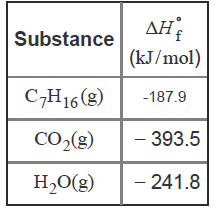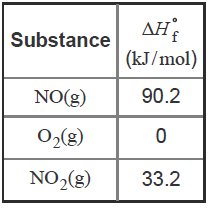# Problem: Part B. The combustion of heptane, C7H16, occurs via the reactionC7H16 (g) + 11 O2(g) → 7 CO2(g) + 8 H2O(g)with heat of formation values given by the following table:Calculate the enthalpy for the combustion of 1 mole of heptane.Express your answer to four significant figures and include the appropriate units.The standard heat of formation, ΔH∘f , is defined as the enthalpy change for the formation of one mole of substance from its constituent elements in their standard states. Thus, elements in their standard states have ΔH°f = 0. Heat of formation values can be used to calculate the enthalpy change of any reaction.Consider, for example, the reaction2 NO(g) + O2(g) ⇌ 2 NO2(g)with heat of formation values given by the following table:∆Hrxn = ∆H°fproducts-∆H°freactants∆Hrxn = 2 (33.2) - 290.2+0∆Hrxn = -114 kJ

###### FREE Expert Solution
82% (345 ratings)View Complete Written Solution
###### Problem Details

Part B. The combustion of heptane, C7H16, occurs via the reaction

C7H16 (g) + 11 O2(g) → 7 CO2(g) + 8 H2O(g)

with heat of formation values given by the following table:Calculate the enthalpy for the combustion of 1 mole of heptane.
Express your answer to four significant figures and include the appropriate units.

The standard heat of formation, ΔHf , is defined as the enthalpy change for the formation of one mole of substance from its constituent elements in their standard states. Thus, elements in their standard states have ΔH°f = 0. Heat of formation values can be used to calculate the enthalpy change of any reaction.

Consider, for example, the reaction

2 NO(g) + O2(g) ⇌ 2 NO2(g)

with heat of formation values given by the following table: